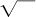NextPrevious

# When did the concept of square root originate?

A square root of a number is a number that, when multiplied by itself, equals the given number. For instance, the square root of 25 is 5 (5 × 5 = 25). The concept of the square root has been in existence for many thousands of years. Exactly how it was discovered is not known, but several different methods of exacting square roots were used by early mathematicians. Babylonian clay tablets from 1900 to 1600B.C.E. contain the squares and cubes of integers 1 through 30. The early Egyptians used square roots around 1700B.C.E., and during the Greek Classical Period (600 to 300B.C.E.) better arithmetic methods improved square root operations. In the sixteenth century, French mathematician René Descartes (1596–1650) was the first to use the square root symbol, called “the radical sign,”.

Close

This is a web preview of the "The Handy Science Answer Book" app. Many features only work on your mobile device. If you like what you see, we hope you will consider buying. Get the App